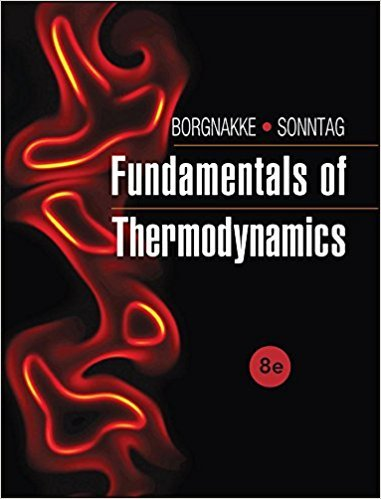×
Get Full Access to Fundamentals Of Thermodynamcs - 8 Edition - Chapter 2 - Problem 56hp
Get Full Access to Fundamentals Of Thermodynamcs - 8 Edition - Chapter 2 - Problem 56hp

×

# Ammonia at 20°C with a quality of 50% and a total mass ofISBN: 9781118131992 58

## Solution for problem 56HP Chapter 2

Fundamentals of Thermodynamcs | 8th Edition

• Textbook Solutions
• 2901 Step-by-step solutions solved by professors and subject experts
• Get 24/7 help from StudySoup virtual teaching assistantsFundamentals of Thermodynamcs | 8th Edition

4 5 1 249 Reviews
16
2
Problem 56HP

Problem 56HP

Ammonia at 20°C with a quality of 50% and a total mass of 2 kg is in a rigid tank with an outlet valve at the top. How much vapor mass can be removed through the valve until the liquid is gone, assuming that the temperature stays constant?

Step-by-Step Solution:

Solution 56HP

Step 1 of 2

We are required to calculate the amount of removed vapor mass.

Step 2 of 2

##### ISBN: 9781118131992

This full solution covers the following key subjects: mass, valve, quality, gone, liquid. This expansive textbook survival guide covers 7 chapters, and 1462 solutions. The answer to “Ammonia at 20°C with a quality of 50% and a total mass of 2 kg is in a rigid tank with an outlet valve at the top. How much vapor mass can be removed through the valve until the liquid is gone, assuming that the temperature stays constant?” is broken down into a number of easy to follow steps, and 48 words. Fundamentals of Thermodynamcs was written by and is associated to the ISBN: 9781118131992. Since the solution to 56HP from 2 chapter was answered, more than 507 students have viewed the full step-by-step answer. This textbook survival guide was created for the textbook: Fundamentals of Thermodynamcs , edition: 8. The full step-by-step solution to problem: 56HP from chapter: 2 was answered by , our top Engineering and Tech solution expert on 08/03/17, 05:05AM.

Unlock Textbook Solution